# Problem: Jeanette is playing in a 9-ball pool tournament. She will win if she sinks the 9-ball from the final rack, so she needs to line up her shot precisely. Both the cue ball and the 9-ball have mass,and the cue ball is hit at an initial speed of . Jeanette carefully hits the cue ball into the 9-ball off center, so that when the balls collide, they move away from each other at the same angle  from the direction in which the cue ball was originally traveling (see figure). Furthermore, after the collision, the cue ball moves away at speed , while the 9-ball moves at speed . For the purposes of this problem, assume that the collision is perfectly elastic, neglect friction, and ignore the spinning of the balls.Find the angle  that the 9-ball travels away from the horizontal, as shown in the figure.Express your answer in degrees to three significant figures.

###### FREE Expert Solution

Let the subscripts c and 9 represent the cue and the 9 balls, respectively.

From the law of conservation of kinetic energy:

$\begin{array}{rcl}{\mathbf{K}}_{\mathbf{i}}& \mathbf{=}& {\mathbf{K}}_{\mathbf{f}}\\ \frac{\mathbf{1}}{\mathbf{2}}{\mathbf{m}}_{\mathbf{c}}{{\mathbf{v}}_{\mathbf{i}}}^{\mathbf{2}}\mathbf{+}\frac{\mathbf{1}}{\mathbf{2}}{\mathbf{m}}_{\mathbf{9}}{{\mathbf{v}}_{\mathbf{i}}}^{\mathbf{2}}& \mathbf{=}& \frac{\mathbf{1}}{\mathbf{2}}{\mathbf{m}}_{\mathbf{c}}{{\mathbf{v}}_{\mathbf{f}}}^{\mathbf{2}}\mathbf{+}\frac{\mathbf{1}}{\mathbf{2}}{\mathbf{m}}_{\mathbf{9}}{{\mathbf{v}}_{\mathbf{f}}}^{\mathbf{2}}\end{array}$

86% (390 ratings)###### Problem Details

Jeanette is playing in a 9-ball pool tournament. She will win if she sinks the 9-ball from the final rack, so she needs to line up her shot precisely. Both the cue ball and the 9-ball have mass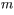,and the cue ball is hit at an initial speed of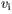. Jeanette carefully hits the cue ball into the 9-ball off center, so that when the balls collide, they move away from each other at the same angle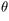from the direction in which the cue ball was originally traveling (see figure). Furthermore, after the collision, the cue ball moves away at speed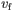, while the 9-ball moves at speed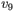.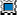For the purposes of this problem, assume that the collision is perfectly elastic, neglect friction, and ignore the spinning of the balls.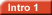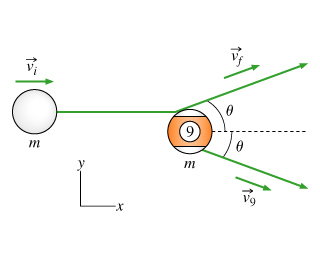Find the anglethat the 9-ball travels away from the horizontal, as shown in the figure.